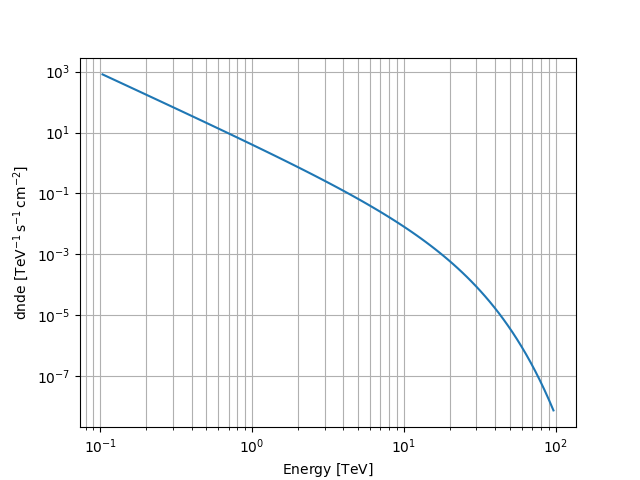# Exponential cutoff power law spectral model used for 3FGL#

This model parametrises a cutoff power law spectrum used for 3FGL.

It is defined by the following equation:

$\phi(E) = \phi_0 \cdot \left(\frac{E}{E_0}\right)^{-\Gamma} \exp \left( \frac{E_0 - E}{E_{C}} \right)$

## Example plot#

Here is an example plot of the model:

from astropy import units as u
import matplotlib.pyplot as plt
from gammapy.modeling.models import ExpCutoffPowerLaw3FGLSpectralModel, Models, SkyModel

energy_bounds = [0.1, 100] * u.TeV
model = ExpCutoffPowerLaw3FGLSpectralModel(
index=2.3 * u.Unit(""),
amplitude=4 / u.cm**2 / u.s / u.TeV,
reference=1 * u.TeV,
ecut=10 * u.TeV,
)
model.plot(energy_bounds)
plt.grid(which="both")## YAML representation#

Here is an example YAML file using the model:

model = SkyModel(spectral_model=model, name="exp-cutoff-power-law-3fgl-model")
models = Models([model])

print(models.to_yaml())

components:
-   name: exp-cutoff-power-law-3fgl-model
type: SkyModel
spectral:
type: ExpCutoffPowerLaw3FGLSpectralModel
parameters:
-   name: index
value: 2.3
-   name: amplitude
value: 4.0
unit: cm-2 s-1 TeV-1
-   name: reference
value: 1.0
unit: TeV
-   name: ecut
value: 10.0
unit: TeV


Gallery generated by Sphinx-Gallery